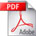# Introduction to Multivariate Regression Analysis

Hippokratia 2010; 14 (Suppl 1): 23-28

EC. AlexopoulosAbstract

Statistics are used in medicine for data description and inference. Inferential statistics are used to answer questions about the data, to test hypotheses (formulating the lternative or null hypotheses), to generate a measure of effect, typically a ratio of rates or risks, to describe associations (correlations) or to model relationships (regression) within the data and, in many other functions. Usually point estimates are the measures of associations or of the magnitude of effects. Confounding, measurement errors, selection bias and random errors make unlikely the point estimates to equal the true ones. In the estimation process, the random error is not avoidable. One way to account for is to compute p-values for a range of possible parameter values (including the null). The range of values, for which the p-value exceeds a specified alpha level (typically 0.05) is called confidence interval. An interval estimation procedure will, in 95% of repetitions (identical studies in all respects except for random error), produce limits that contain the true parameters. It is argued that the question if the pair of limits produced from a study contains the true parameter could not be answered by the ordinary (frequentist) theory of confidence intervals. Frequentist approaches derive estimates by using probabilities of data (either p-values or likelihoods) as measures of compatibility between data and hypotheses, or as measures of the relative support that data provide hypotheses. Another approach, the Bayesian, uses data to improve existing (prior) estimates in light of new data. Proper use of any approach requires careful interpretation of statistics .